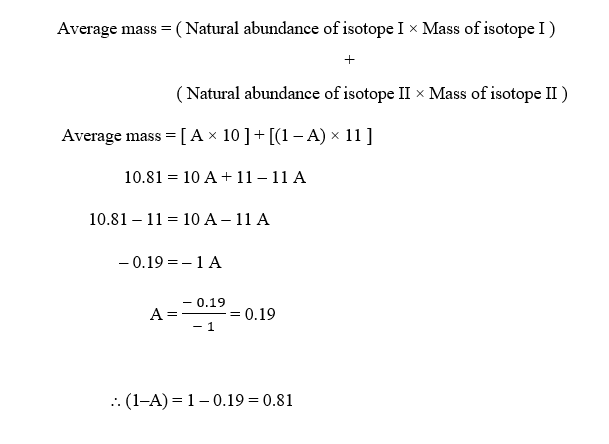# Almost all boron atoms are found in two forms: boron-10 and boron-11. Both isotopes behave alike chemically and are useful in fireworks (green color), in the antiseptic boric acid, and in heat-resistant glass. If the average atomic mass of boron is 10.81 amu, which boron isotope must be more abundant in nature? How do you know?

Question
4 views

Almost all boron atoms are found in two forms: boron-10 and boron-11. Both isotopes behave alike chemically and are useful in fireworks (green color), in the antiseptic boric acid, and in heat-resistant glass. If the average atomic mass of boron is 10.81 amu, which boron isotope must be more abundant in nature? How do you know?

check_circle

Step 1

Given,

Boron atoms are found in two forms: boron-10 and boron-11

Average mass of B = 10.81 amu

Step 2

Since, the value 10.81 amu is a lot closer to 11 amu than it is to 10 amu, so there must be more of boron-11 than boron-10.

To confirm this, we can also do a simple calculation to find the natural abundance of boron-11 and boron-10.

Let us assume :

Atomic mass of 10B (isotope I) = 10 amu

Atomic mass of 11B (isotope II) = 11 amu

Natural abundance of 10B (isotope I) = A

Natural abundance of 11B (isotope II) = (1 – A)

The average mass of B is expressed as:Since natural abundance is expressed as percentage, ther...

### Want to see the full answer?

See Solution

#### Want to see this answer and more?

Solutions are written by subject experts who are available 24/7. Questions are typically answered within 1 hour.*

See Solution
*Response times may vary by subject and question.
Tagged in